Data visualization is one of the most effective ways to deliver the key message to the audience, as it helps explore the hidden patterns in the datasets. There are numerous tools available to generate plots. Even specialized tools are available nowadays, such as Tableau or Power BI specially designed for data visualization. As of today, there are two great programming tools available for data analytics, which are Python and R. These programming languages also have various wonderful user written libraries for generating wonderful visualizations.

Here, in this blog series, we are going to explore the most fundamental libraries for generating publication ready visualizations using Python programming language. These libraries are Matplotlib and Seaborn.

Matplotlib: The Matplotlib is the base, i.e., a low-level interface for generating static, animated, and interactive visualizations using an object-oriented approach.

Seaborn: The Seaborn library is a high-level (low code) interface for generating beautiful, specialized statistical plots.

In this visualization blog series, we will start with exploring the nuts and bolts of the Matplotlib library. We will try our best to learn the following:

• Basics attributes and methods of matplotlib
• How this library builds a plot from scratch
• How to customize plots
• How to arrange them in a grid
• How to interchangeably use Matplotlib, Pandas and Seaborn

Once we have a better idea of the Matplotlib library, then we will proceed with both Matplotlib and Seaborn for generating more complex plots.

## What will be covered in this data visualization blog series:

In the current article, we will get familiar with the Matplotlib library and then learn how to build a line plot from scratch.

## Article Outline

• Introduction
• Importing libraries
• Line plot
Basics of plotting using a line plot
Line plot using real-world dataset
Line plot customization
Plotting time series data using twin axis line plot

## Importing libraries

Before proceeding to the visualization part, first we need to import numpypandasmatplotlib and seaborn libraries.

If you haven’t installed them, then first install them using pip or conda in your existing environment. Once the installation is complete, you can use the following code to import them.

``````import numpy as np
import pandas as pd
import matplotlib.pyplot as plt
import seaborn as sns``````

## Concept of subplots

I will start with the most important line of code that we will be using for generating every plot. There are multiple ways one can generate plot using matplotlib. I will recommend learning at least one method of doing it. I mostly prefer the matplotlib’s subplots( ) method.

In a simple sentence, a subplot is a method for defining a canvas where you would like to draw geometrics (line, circles and so on). The subplots object can be used to arrange multiple plots in a grid manner. You can define and alter attributes of the plot and its various properties, for example colour, shape, size.

The first thing in matplotlib is defining the plotting space using the plt.subplots( ) method.

The subplots( ) method takes various arguments, but mostly we supply the following:

• figsize: It defines the figure size in inches (takes as tuple), where the first argument is for width and the second argument is for height, i.e., (width, height).
• nrows: It denotes the number of rows for the grid (where we would like to add our plot).
• ncols: It denotes the number of columns for the grid (where we would like to add our plot).

For, example (see below code) here we have set the figure size (width = 16 and height = 7) in inches. Next, we supplied that we need a grid of 4 rows and 4 columns, which can take in total 16 plots. We require this type of grid when we need to add multiple plots in a single plot in a grid manner.

When we run the following code, it will display the following text (returned objects) and a plot of 16 subplots (grids).

Let’s understand the text output. The text output contains two parts:

• figure object: The first part of the text contains the figure object, which shows the Figure size and also a text stating it contains 16 Axes
• axes object: The second part of the text contains an array (list of list) of 16 axes (AxesSubplots), as we requested by supplying the nrows and ncols in subplots( ) method.
``````# Figure and axes
plt.subplots(figsize = (16, 7), nrows = 4, ncols = 4)``````

So for generating and arranging various plots we mostly require the axes object, where we impose our data using different geometric features such as lines or bars and so on.

Let’s understand the above figure (or refer to the array containing Axes subplots: <Axessubplots:>) to understand the axes object. Each square plot (subplot) in the above 4 by 4 grid is represented using a particular address. As python is zero (0) indexed based language; thus the above image (grid) can be translated into the following numerical grid (as shown below).

[0,0] [0,1] [0,2] [0,3]
[1,0] [1,1] [1,2] [1,3]
[2,0] [2,1] [2,2] [2,3]
[3,0] [3,1] [3,2] [3,3]

• Here it is a 4 by 4 grid same as above image but represented using row and column numbers
• The number in the square brackets denote row and column number i.e, [row, column]

For example, the last image in the bottom-right corner is situated at row 3 and column 3 position, i.e., [3,3].

Now you will be wondering why we need to translate it into a numbered grid?

Because when we would like to add several plots into the defined grid we need the specific address, i.e., [row, column].

Saving fig and axis objects

So, now we understand that plt.subplots( ) returns two object (i) figure and (ii) axes.

To use them, we can save the returned figure and axes objects to `fig` and `ax` variables.

Note: When you save them to fig and ax, the output still generates the subplots figure as shown below.

``````# Saving the returned figure and axes objects
fig, ax = plt.subplots(figsize = (16, 7), nrows = 4, ncols = 4)``````

Creating a single blank plot using subplot

We have learned to create subplots using the subplots( ) method, but we will come back to this later.

Currently, we will just focus on a single axes object (single plot). Let’s create a single plot object using subplots( ). To accomplish this, we need to supply the figsize argument, which by default generates a single plot, as shown below. We will save the retuned figure and axes object to fig and ax respectively.

``fig, ax = plt.subplots(figsize = (12, 6))``

Checking the type of figure object

You might be curious to know what would be the object type for fig and ax. If we check the type of figure (fig) object, it will return a `matplotlib.figure.Figure`.

``type(fig)``

matplotlib.figure.Figure

Checking the type of axes object

Similarly, if we check the type of axes (ax) object, it will return a `matplotlib.axes._subplots.AxesSubplot`.

``type(ax)``

matplotlib.axes._subplots.AxesSubplot

Let’s check the figure dpi

We can also check the default image dpi for the figure object. The matplotlib by default set it to 72 dpi.

``````# Check default dpi
fig.dpi``````

72.0

Let’s check the matplotlib figure size

We can apply the get_size_inches( ) method, which will return the figure size in inches. The output is the same size (12, 6) as the argument we set inside the subplots( ) method.

``fig.get_size_inches()``

array([12., 6.])

Checking the axes object

The figure object (fig) also contains the Axes Subplot information. We can use the fig.axes to identify the object it contains. It is a AxesSubplot object.

``fig.axes``

[<AxesSubplot:>]

Whether fig.axes object is same as ax

Let’s check whether the first element of fig.axes and ax object is same. The output shows True,indicating they are the same. Though we can access the axes object from fig, still we will extensively use the saved ax object for generating plots.

``fig.axes is ax``

True

Children associated with axes object (ax)

Let’s check what this axes (ax) object contains. We can print its elements using the get_children( ) method.

The axes (ax) object contains the following:

• 4 Spines object (Spines are boundary lines across the plot)
• 2 axis objects (x and y-axis)
• Text related to tick labels
• Matplotlib rectangular patch object
``ax.get_children()``

Checking the Spines

Let’s check the four Spines. We can access the spines using ax.spines, but to get the Spines position we need to iterate through it using a for loop. The output shows the four spines are located in the left, right, bottom and top of the plot.

``````for s in ax.spines:
print(s)``````

left
right
bottom
top

Properties associated with each plot objects

The axes object contains various properties that help in formulating the plot. We can alter these properties as per our requirement to generate the required plot.

For, example, let’s see what properties the xaxis has. We can apply the properties( ) method on xaxis object to see the associated properties and their default values.

Note: We will later see that there are two types of method associated with matplotlib objects. The first type can be used to see the associated values (get methods) and the second type of method (set methods) can be used to alter them.

``ax.xaxis.properties()``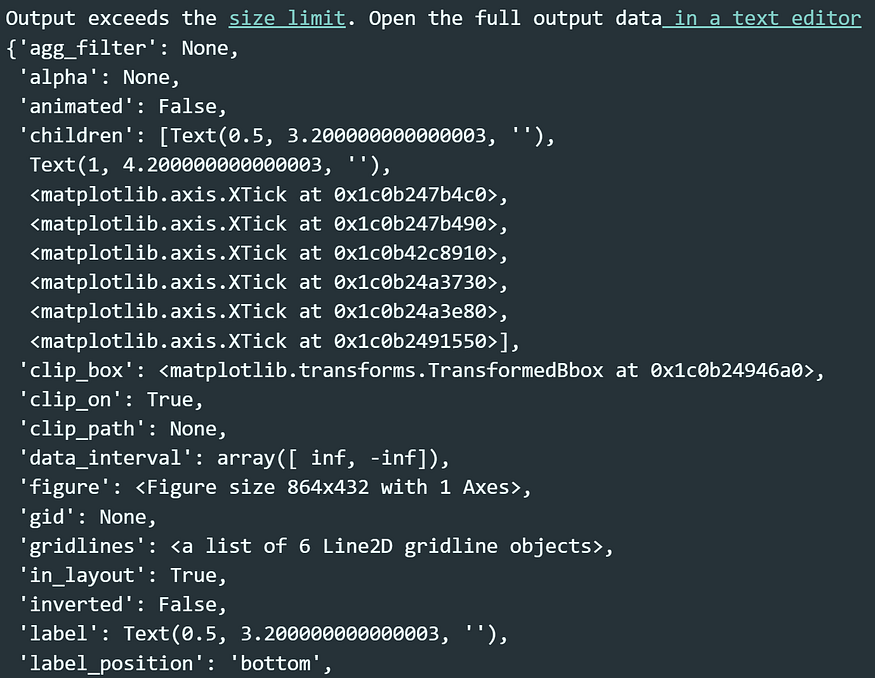Few properties of the xaxis object

# Basics of matplotlib with a line plot

What is a line plot?
A line plot is a way to display data along a number line.

Before we proceed with a real-world dataset, first let’s understand how matplotlib builds a line plot step by step.

To generate a line plot, we need to go through the following steps:

Step 1: Import the Line2D method from matplotlib.lines
Step 2: Generate x-axis values. Here we used the np.linspace to generate 10 numeric values.
Step 3: Generate y values. Here, we would like to plot two lines. Thus, we have added to list of values to y1 and y2.
Step 4: The next step is to add the x and y values in the Line2D( ) method, as we want to generate a 2D line plot. We need to supply y1 and y2 separately to generate two separate lines. Then we need to save them into two separate variables l1 and l2. We can also add the `label` identifier that will help us later to generate the legend.

``````# Import the Line2D method
from matplotlib.lines import Line2D
# Generate x-axis values (10 numbers between 1 and 20)
x = np.linspace(start = 1, stop = 20, num = 10)
print(x)
# Generate y values for two separate lines
y1 = [2, 3, 9, 10, 11, 15, 17, 5, 9, 1]
y2 = [1, 2, 8, 9, 9, 10, 11, 4, 8, 1]
# Adding x and y values to Line2D method
l1 = Line2D(x, y1, label = "line1")
l2 = Line2D(x, y2, label = "line2")``````

[ 1. 3.11111111 5.22222222 7.33333333 9.44444444 11.55555556 13.66666667 15.77777778 17.88888889 20.

Adding lines to axes object

Once we have the Line2D objects, i.e., `l1` and `l2`, the next step is to impose them over the axes object (previously generated) using add_line( ) method. We also need to rescale the plot using the ax.autoscale( ) to view the lines.

The next step is to call the `fig` object to view the plot.

``````# adding lines to ax object
ax.autoscale()
# Plotting the figure object
fig``````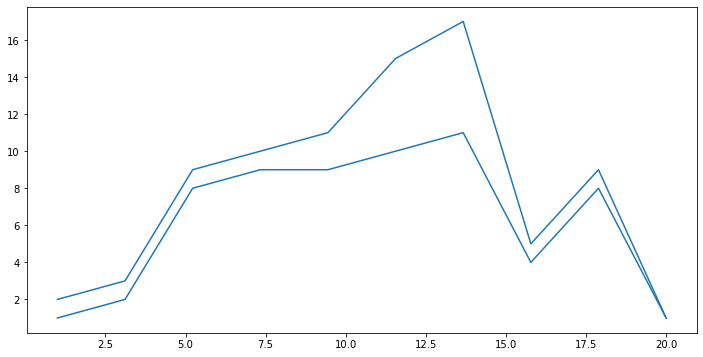First line plot with two separate lines

Checking the axes object

Now we have added the lines to our axes (ax) object. Let’s make a query to know whether the axes (ax) object contains both the lines. We can query this by adding .lines attribute to our axes (ax) object.

The output clearly shows that the axes (ax) object now contains 2 lines.

``````# Checking axes object
ax.lines``````

<Axes.ArtistList of 2 lines>

Changing a line’s colour

Now we have confirmed that the axes (ax) object contains two lines, we can alter their properties to make it more appealing.

Let’s change the color for the first line to “red”. This can be accomplished by the following steps:

• Step 1: access the first line using ax.lines
• Step 2: use .set_color( ) method and supply the color name as string
• Step 3: display the `fig` object to view the change
``````# Changing colour of line no. 0 (zero)
ax.lines.set_color("red")
fig``````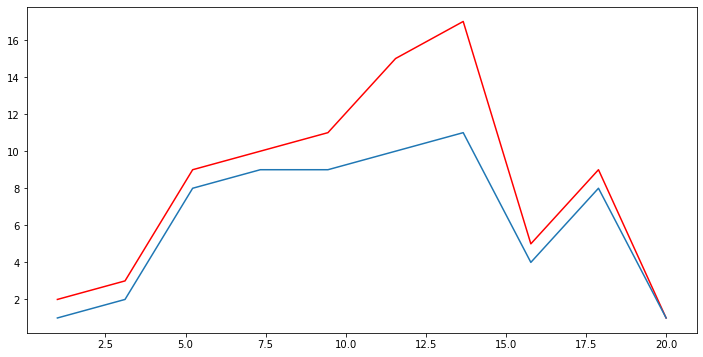Changing the first line’s colour to red

Customizing lines

Let’s change a few properties of the line 1. We can use the .set_alpha( ) to increase/decrease transparency of the lines. The alpha value ranges 0 to 1. In addition, we can use the .set_linestyle( ) method to change the style. Here, we set it to “dashed” version.

``````# Making line 1 transparent and changing line type to "dashed"
ax.lines.set_alpha(0.4)
ax.lines.set_linestyle("dashed")
fig``````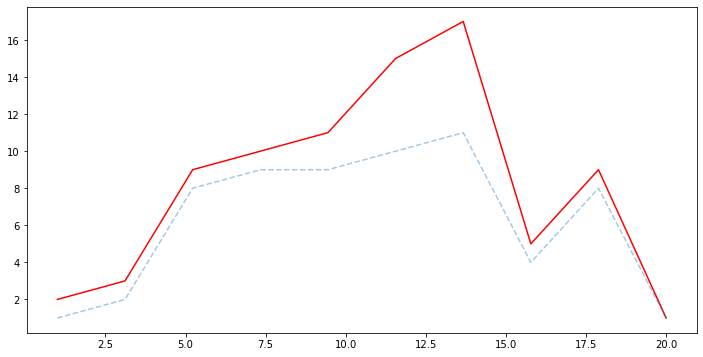Making line two transparent and transparent

Adding and customizing markers

Next, we will add markers to the values using .set_markers( ) method. For line 1 we will use a triangular marker (`“`^`”`) and for line 2 a star shaped marker (`“`*`”`). In addition, we will change the marker size to 10 using .set_markersize( ) method.

``````# Adding markers and changing markers' size
ax.lines.set_marker("^")
ax.lines.set_marker("*")
ax.lines.set_markersize(10)
ax.lines.set_markersize(10)
fig``````

Changing x-axis limit

We can also extend the axis range. Here I have extended the x-axis range (from 20 to 25) using .set_xlim(start, end) method.

``````# Change the x-axis limit
ax.set_xlim(0, 25)
fig``````

Even though the plot looks beautiful, still we need to add an identifier to each line. To do so, we need to call the .legend( ) method on the axes (ax) object, which will add the legend based on the labels we supplied earlier.

``````# Adding plot legend
ax.legend()
fig``````

I hope now you understand the basics of matplotlib and how it creates a plot from scratch. Though this way of generating plot is systematic, but it requires too much code and time.

As a data scientist or analyst, we need a fast, low code approach for generating plots using real-world datasets.

We will proceed to the low code approach step by step 😃

# Line Plot using Real-World Data

For the current plot, we are going to use tips dataset.

Source:
Bryant, P. G. and Smith, M. A. (1995), Practical Data Analysis: Case Studies in Business Statistics, Richard D. Irwin Publishing, Homewood, IL.

The Tips data contains 244 observations and 7 variables (excluding the index). The variables descriptions are as follows:

bill: Total bill (cost of the meal), including tax, in US dollars
tip: Tip (gratuity) in US dollars
sex: Sex of person paying for the meal (Male, Female)
smoker: Presence of smoker in a party? (No, Yes)
weekday: day of the week (Saturday, Sunday, Thursday and Friday)
time: time of day (Dinner/Lunch)
size: the size of the party

Let’s load the tips dataset using pandas read_csv( ) method and print the first 5 observations using head() method.

``````# Reading the dataset

Creating a line plot

Now we have loaded the dataset, it is time for generating a line plot. Here we are going to plot the total_bill and tip columns using two lines imposing over an axes object.

To generate the plot, we need to go through the following steps:

• Step 1: First generate a subplots object and save the returned figure and axes object in fig and ax respectively.
• Step 2: The next step is to impose a plot on the axes object (ax). Here, first we supplied the total_bill values in the plot( ) method and imposed it over axes object ax.plot( ). By default, ax.plot( ) generates a line plot. You can also supply the x-axis values, but here we ignored it. So, if we do not provide the x-axis values, it will take the index values from tips dataset as x-axis values. Next, we need to provide the dataset name and an identifier for the line (label = “Total bill”).
• Step 3: Next we need to add the next y2 values, i.e., our `tip` column to add another line on the existing plot using ax.plot( ) method. Here, we added another identifier (label = “Tips”).
• Step 4: The next step is to call the legend( ) method on the axis object (ax), which generate the identifiers for the lines.
• Step 5: Next, we modified the x and y-axis labels and their size using set_xlabel( ) and set_ylabel( ) methods.
``````fig, ax = plt.subplots(figsize = (16, 7)) # we can add dpi = 300
# Add two lines
# if x-axis values were not provided, x-axis will take index values # from pandas DataFrame
# Add y-values for total_bill
ax.plot("total_bill", data = tips, label = "Total bill")
# Add y-values for tips
ax.plot("tip", data = tips, label = "Tips")
ax.legend()
# Add axis labels
ax.set_xlabel("Index values of tips", size = 14)
ax.set_ylabel("Total bill/tip amount", size = 14)``````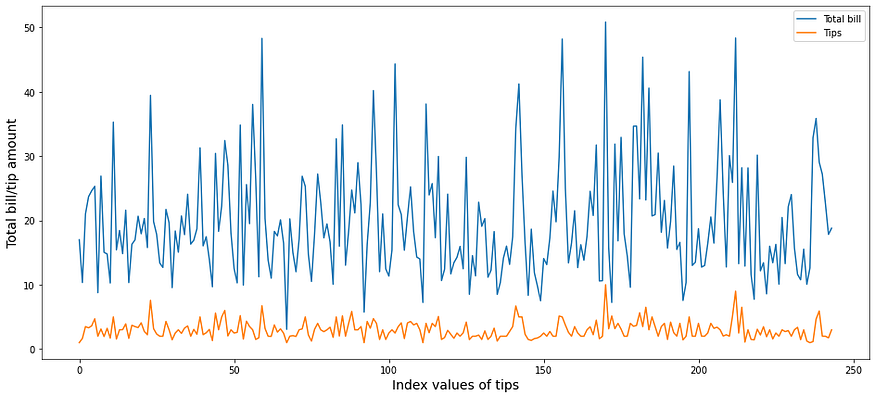First line plot using tips dataset

Customizing the line plot

Next, we will customize the plot to make it beautiful.

• Here, we set the line 0 and 1 marker’s shape to circle using set_marker(“o”)
• We can set the marker interval using set_markevery( ). Here, we set it to an interval of 5.
• Also, let’s set the marker face colour to white colour using set_markerfacecolor(“white”)
``````# Adding "o" markers
ax.lines.set_marker("o")
ax.lines.set_marker("o")
# Setting marker's interval
ax.lines.set_markevery(5)
ax.lines.set_markevery(5)
# Setting marker's colour
ax.lines.set_markerfacecolor("white")
ax.lines.set_markerfacecolor("white")``````

Adding \$ sign on y-axis tick-labels

Next, we will perform some customization on the y-axis tick labels. We are going to add a dollar (\$) sign in front of y-axis tick labels. To do so, we need to import the StrMethodFormatter from matplotlib.ticker module.

• First, we need to create a string formatter object StrMethodFormatter(“\${x: .0f}”) to add the dollar sign. The code {x: .0f} refers to the zero-decimal place after the number.
• Next, adding the formatter object to y-axis using the set_major_formatter( ) method and saving it into mftvariable.
• Further, we have customized both the x and y-axis tick parameters using the tick_params( ) method, where we set the tick label size to 16 and label color to red.
``````# Import StrMethodFormatter
from matplotlib.ticker import StrMethodFormatter
# Use string formatter
mft = StrMethodFormatter("\${x: .0f}")
# Set string formatter
ax.yaxis.set_major_formatter(mft)
# Setting tick labels to size 12 and color "red"
ax.tick_params(axis = "both", labelsize = 16, labelcolor = "red")``````

Saving the line plot as an image file

The last step after plot customization is to save the figure. To do so, we need to go through the following steps:

• First, let’s say we want to save the figure in our current directory but inside a new directory named “images”. To create a new directory or to check whether the directory is already available, we need to use the os library and a try and except clause.
• We can save a figure by applying the savefig( ) method on the figure object (fig). Here we have saved the figure by providing a name “line_chart.png” and also set the resolution to 300 dpi.
``````# Use os library
import os
# Use try and except
# The following code will Check whether the `images` folder is
# available in your current directory and if `images` folder is not
# available, create a new one
try:
os.mkdir('images')
except:
print('Image dir already exist!')
# Saving the current figure
fig.savefig("images/line_chart.png", dpi = 300)``````

# Plotting Time Series Data using Twin Axis Line Plot

Let’s begin with a time series data visualisation using various plotting mechanisms. Here we will use three styles to generate the same plot, which are:

• Matplotlib style
• Pandas style
• Seaborn style

Here we are going to use the sales dataset which contains observation date, a dummy company’s Sales, AdBudget and GDP figure in some arbitrary unit. Our goal is to plot and compare Sales with AdBudget over the years.

Let’s load the data using pandas read_csv( ) and we also set the parse_dates = True so that it will convert a date time column to pandas date time object while reading the dataset. Further, we supplied index_col = “Date” to set the `Date` column as pandas DataFrame index. Setting datetime column as index is always preferred while dealing with time series data.

``````sales_data = pd.read_csv("datasets/Sales_dataset.csv",
parse_dates=True,
index_col = "Date")

A twin axis plot has a common x-axis and two y-axes for plotting two separate column values. This type of plot is useful when we want to compare two variables of different unit or scale.

Here are a few scenarios where a twin-axis plot might be appropriate:

• Comparing Co2 emission and temperature rise/fall over the year.
• Comparing yearly ads budget and sales.

## Plotting a twin-axis plot using matplotlib library [matplotlib style]

Let’s create a twin axis plot.

Generating the first-half of the plot

I have divided the plot generation to three parts to simplify the code understanding. In the first half, we will plot the Sales data. To generate the plot, follow the steps:

• Step 1: The first step is as usual, creating a subplots object and saving the figure and axes object into fig and ax.
• Step 2: Next apply the plot method on the axes object (ax). Then supply the index of the sales data to x and Sales column to y argument position, followed by dataset namecolor of the line and a label identifier which will be used to generate the legend. We saved the first half of the plot in l1 variable that we later use to generate the legend.
• Step 3: Next step is to add labels to and y-axis using set_xlabel( ) and set_ylabel( ). We also modified the y-axis tick parameters using tick_params( ) method.
``````################################################
# Part 1: Add sales to y1 axis
################################################
fig, ax = plt.subplots(figsize = (10, 5)) # dpi = 300
l1 = ax.plot(sales_data.index,
"Sales",
data = sales_data,
color = "blue",
label = "Sales")
# Set the axis label
ax.set_xlabel("Time (years)")
ax.set_ylabel("Sales", color = "blue")
# Set tick parameters
ax.tick_params(axis = 'y', colors = "blue")``````

Generating the second-half of the plot

To add the second half of the plot, follow the steps:

• Step 1: In the second half, we are going to plot the AdBudget variable in another y-axis, i.e., y2-axis on the right side of the current plot. For that, we need to specify that we want another axis to plot y2 using the ax.twinx( ) method and save the new axis to ax2.
• Step 2: Next, using the same .plot( ) method we are going to add the AdBudget column to the new ax2-axis. We saved the second half of the plot in l2 variable.
• Step 3: We here also altered the ylabel and tick parameter properties.
``````#################################################
# Part 2: Add AdBudget to y2 axis
################################################
ax2 = ax.twinx() # adding an axis y2-axis with common x-axis
l2 = ax2.plot(sales_data.index,
data = sales_data,
color = "red",
# Set the y-axis label and modify the tick parameters
ax2.set_ylabel("AdBudget", color = "red")
ax2.tick_params(axis = 'y', colors = "red")
fig``````

The next step is to add the legend. For normal single axis plot it is straightforward but in twin axis plot it is little bit tricky. Here, we will learn two ways of adding legend to twin-axis plot (applicable for matplotlib style plotting).

• First, we need to add the l1 and l2 objects and save it to a new variable lines.
• The next step is to use a list comprehension to iterate though lines and extract the labels using get_label( ) method
• Finally, supply the lines and labels (labs) to legend( ) method to generate a legend for the twin axis plot.
``````################################################
# Part 3 (A): Adding legends
################################################
lines = l1 + l2
# Use list comprehension to get labels
labs = [l.get_label() for l in lines]
ax.legend(lines, labs)
fig
``````

The legend can be added using another technique. The legend( ) method takes the handles argument, where we can supply the list of line elements of each x-axis (ax and ax2) and manually supply the labels. It will produce the same result as shown above.

``````################################################
# Part 3 (B): Adding legends
################################################
ax2.legend(handles = [a.lines for a in [ax, ax2]],
labels = ["Sales", "AdBudget"])``````

Full code for the twin-axis plot

Here is the full code for generating a twin-axis plot (Matplotlib way).

``````################################################
# Part 1: Add 'sales' to y1 axis
################################################
fig, ax = plt.subplots(figsize = (10, 5)) # dpi = 300
l1 = ax.plot(sales_data.index,
"Sales",
data = sales_data,
color = "blue",
label = "Sales")
# Set the axis label and tick parameters
ax.set_xlabel("Time (years)")
ax.set_ylabel("Sales", color = "blue")
ax.tick_params(axis = 'y', colors = "blue")
#################################################
# Part 2: Add 'AdBudget' to y2 axis
################################################
ax2 = ax.twinx()
l2 = ax2.plot(sales_data.index,
data = sales_data,
color = "red",
# Set the axis label and tick paraemeters
ax2.set_ylabel("AdBudget", color = "red")
ax2.tick_params(axis = 'y', colors = "red")
################################################
# Part 3: Adding legends
################################################
lines = l1 + l2
labs = [l.get_label() for l in lines]
ax.legend(lines, labs)
################################################
# Part 4: Saving the figure
################################################
fig.savefig("images/twinaxis_plot.png", dpi = 300)``````

## Plotting a twin-axis plot using pandas plot method [pandas style]

In addition to Matplotlib, we can directly apply the plot( ) method on our pandas DataFrame. Pandas also allows quick plotting using the Matplotlib in the backend.

To generate the same plot in pandas way, we need to filter out the columns that we want to add as a line in the plot canvas.

Here, we have filtered out the Sales and AdBudget columns using the DataFrame .loc operator and saved in df variable. Now this data frame contains two columns, Sales and AdBudget with Date as index.

``````df = sales_data.loc[:, ["Sales", "AdBudget"]]

Plotting the twin-axis plot

The pandas way of generating the plot requires minimum number of code lines. To generate the plot, we need to go through the following steps:

• Step 1: The first step is as usual, creating a subplots object and saving the returned figure and axes object into fig and ax.
• Step 2: Apply the plot( ) method over the df DataFrame as df.plot( ). This will take the index from the `df` DataFrame and use it as x-axis values, and columns as y-axis values. We need to separately tell the plot( ) method that which column of the DataFrame (df) will be used for secondary y-axis, i.e.y2, here the y2 is AdBudget. Moreover, we also need to supply the axes object (ax) over which we would like to impose our line geometrics.
• Step 3: The plot customization by adding x and y labels and changing tick parameter properties are same.
• Step 4: To change the secondary y-axis (y2) label and parameters, we need to use the right_ax.set_label( ) and right_ax.tick_params( ) from the axes (ax) object.
``````# Add sales to y1 axis
fig, ax = plt.subplots(figsize = (10, 5))
ax = ax,
color = ["blue", "red"])
# Set the left axis label and tick parameters
ax.set_xlabel("Time (years)")
ax.set_ylabel("Sales", color = "blue")
ax.tick_params(axis = 'y', colors = "blue")
# Set the right axis label and tick parameters
ax.right_ax.set_ylabel("AdBudget", color = "red")
ax.right_ax.tick_params(axis = 'y', colors = "red")``````

## Plotting a twin-axis plot using seaborn library [seaborn style]

The plotting mechanism using seaborn library is similar to the process used for matplotlib styled plotting. The only difference is here we need to use the lineplot( ) method from seaborn (sns) library.

``````# Defining the subplots object
fig, ax = plt.subplots(figsize = (10, 5))
################################################
# Part 1: Add 'sales' to y1 axis
################################################
sns.lineplot(x = sales_data.index,
y = "Sales",
data = sales_data,
color = "blue",
ax = ax)
# Set the axis label and tick parameters
ax.set_xlabel("Time (years)")
ax.set_ylabel("Sales", color = "blue")
ax.tick_params(axis = 'y', colors = "blue")
#################################################
# Part 2: Add 'AdBudget' to y2 axis
################################################
ax2 = ax.twinx()
sns.lineplot(x = sales_data.index,
data = sales_data,
ax = ax2,
color = "red")
# Set the axis label and tick parameters
ax2.set_ylabel("AdBudget", color = "red")
ax2.tick_params(axis = 'y', colors = "red")
################################################
# Part 3: Adding legends
################################################
ax2.legend(handles = [a.lines for a in [ax, ax2]],
labels = ["Sales", "AdBudget"])``````